# HTML5 Canvas : Graphics

## On the understanding of graphics

When one or more paths intersect to produce a closed loop, a graph will be formed. Therefore, the basic element of the figure is the path, and drawing the figure in canvas is to close the path, and then render the figure by tracing or filling the path area.

## Closed path

Closure method:

``````//Create a path from the current point to the start point
context.closePath();
``````

A closed path is a path that creates the end and start points in the current path. Use an example in the Path to do a close test...

``````var canvas=document.getElementById("myCanvas");
var ctx=canvas.getContext("2d");
ctx.beginPath();
ctx.moveTo(20,20);
ctx.lineTo(50,20);
ctx.arcTo(150,20,150,70,50);
ctx.lineTo(150,150);

//Manual closing or
ctx.lineTo(20,20);
//Auto close
ctx.closePath();

ctx.stroke();
``````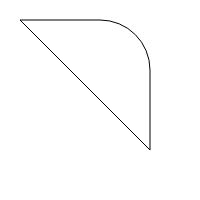Thus, we can draw some basic figures through closed path!

## Draw a rectangle

``````var canvas=document.getElementById("myCanvas");
var ctx=canvas.getContext("2d");
ctx.beginPath();
ctx.moveTo(20,20);
ctx.lineTo(180,20);
ctx.lineTo(180,100);
ctx.lineTo(20,100);
ctx.closePath();
ctx.stroke();
``````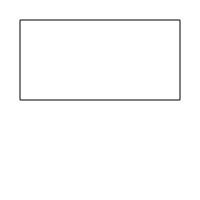## Draw triangle

``````var canvas=document.getElementById("myCanvas");
var ctx=canvas.getContext("2d");
ctx.beginPath();
ctx.moveTo(100,20);
ctx.lineTo(30,100);
ctx.lineTo(170,100);
ctx.closePath();
ctx.stroke();
``````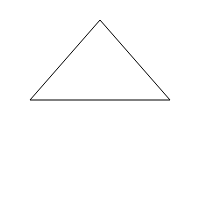## Draw a circle

``````var canvas=document.getElementById("myCanvas");
var ctx=canvas.getContext("2d");
ctx.beginPath();
ctx.arc(100,100,50,0,2*Math.PI);
ctx.stroke();
``````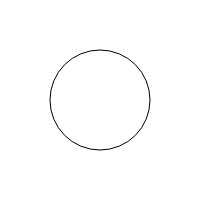## Fill path

Filling method:

``````//Fills the current drawing (path). The default color is black.
context.fill();
``````

If the path is not closed, the fill () method adds a line from the end of the path to the start to close the path and then fill the path.

``````var canvas=document.getElementById("myCanvas");
var ctx=canvas.getContext("2d");
ctx.beginPath();
ctx.moveTo(100,20);
ctx.lineTo(30,100);
ctx.lineTo(170,100);
ctx.fill();
``````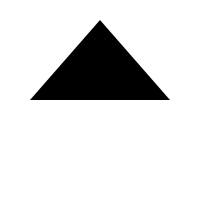# You May Also Like